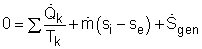Ch 6. Entropy Multimedia Engineering Thermodynamics Entropy Tds Relations EntropyChange IsentropicProcess IsentropicEfficiency EntropyBalance (1) EntropyBalance (2) ReversibleWork
 Chapter 1. Basics 2. Pure Substances 3. First Law 4. Energy Analysis 5. Second Law 6. Entropy 7. Exergy Analysis 8. Gas Power Cyc 9. Brayton Cycle 10. Rankine Cycle Appendix Basic Math Units Thermo Tables Search eBooks Dynamics Fluids Math Mechanics Statics Thermodynamics Author(s): Meirong Huang Kurt Gramoll ©Kurt GramollTHERMODYNAMICS - THEORY Entropy Balance for Control Volumes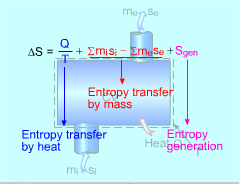Entropy Transferred by Heat and Mass in Control Volume A control volume permits both energy and mass to flow through its boundaries. The entropy balance for a control volume undergoing a process 1-2 can be expressed as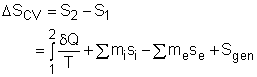or in the rate form, as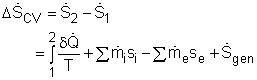where i and e denote inlet and exit, respectively. The above entropy balance relation states that the entropy change of a control volume undergoing a process 1-2 equals the sum of the entropy transfer by heat, the net entropy transfer by mass, and the entropy generation in the control volume. Analysis of a Control Volume at Steady State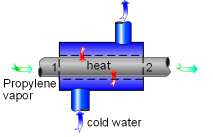Propylene Flows Steadily through a Heat Exchanger Most control volumes encountered in practice, such as nozzles, turbines and compressors operate at steady state. Hence, there is no entropy change in the control volume. That is,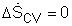The entropy balance in this case is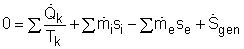In engineering analyses, the mass balance, energy balance, and the entropy balance often must be solved simultaneously. Recall, the mass balance and energy balance are,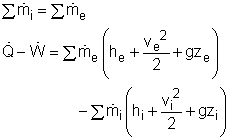One-inlet-one-exit Control Volumes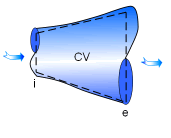A one-inlet-one-exit Nozzle Many applications involve a one-inlet-one-exit control volume at steady state, such as a nozzle. The three balance equations for this case are,       Mass Balance: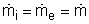Energy Balance: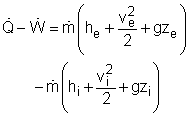Entropy Balance: